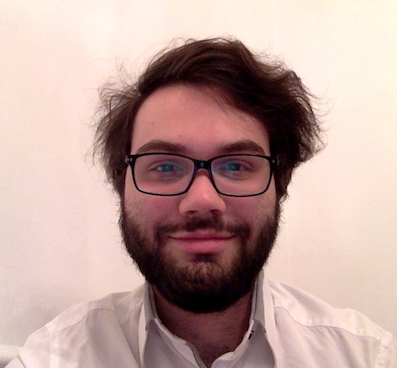# Emmanuel Caron

I am Assistant Professor at Avignon University, affiliated to the Laboratoire de Mathématiques d'Avignon.

My research focuses on the study of statistical models in the case where the random variables are dependent.

During my thesis, I considered the linear regression model assuming that the errors are dependent. Under the very general conditions of a Central Limit Theorem proven by Hannan (1973), I established several consistency results for the estimator of the asymptotic covariance matrix of the least squares estimator. Then I proposed a modification of the usual Fisher tests in order to correct the type I error rate in the case where the observations are correlated. To apply these results on datasets, an R package called slm is available on the CRAN. It contains a set of data driven methods for the linear model in the dependent case.

Next, I studied the nonparametric regression model via a model selection approach, in the case where the error process is a Gaussian short memory dependent sequence. In this context, I established an oracle inequality for the minimal risk estimator among a collection of models. I now consider the case where the error process is a Gaussian long memory dependent sequence.

My research is divided into two parts:

• For the nonparametric regression model, it is necessary to generalize the results when the error process is dependent and non-Gaussian. In collaboration with Antoine Marchina (Université de Paris), we study recent works on concentration inequalities which are likely to be extended to the dependent case. This provides a solid line of research allowing us to focus on the problems of model selection in the dependent case.
• The second part concerns results of the statistical learning theory. In collaboration with Stéphane Chrétien (Université Lumière Lyon 2), we study a non-asymptotic approach for the double descent phenomenon .

### Research interests

• Nonparametric regression
• Model selection
• Slope heuristic
• Statistical learning
• Double descent
• Stationary processes
• Dependence
• Time series
• Spectral density
• Long memory
• Linear regression model
• Hypothesis testing

### ContactAvignon Université
Laboratoire de Mathématiques d'Avignon
74 Rue Louis Pasteur, 84029 Avignon, France

E-mail: emmanuel dot caron-parte at univ-avignon dot fr